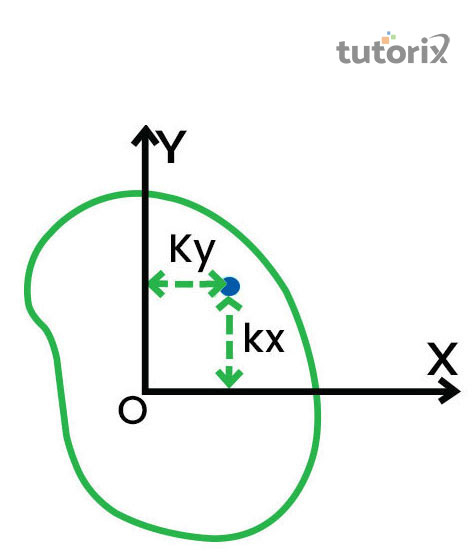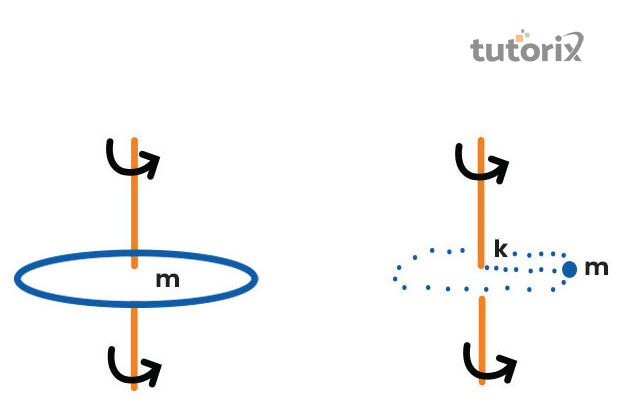## Introduction

The radius of gyration of an object about the axis of its rotation is generally defined as the radial distance. This distance is associated with a point which would have a moment of inertia. This moment of inertia would be equal to the actual mass distribution of the particular body, in case the total mass of the body were to be concentrated at that point.

The radius of the gyration is useful in various subjects throughout the daily course of life, especially in the field of engineering. In real life, this is used to study the rigidity of a column. The radius of gyration is also termed gyroradius.

## What do you mean by Radius of gyration?

The radius of gyration of a body often represents the moment of inertia of a particular body around its particular axis. It can however be defined differently, thus it is to be noted that the radius of gyration can be considered as a hypothetical distance from the centroid of an object (Shaheen et al. 2018).

This centroid is associated with the area of cross-section which is imagined to be concentrated at a particular point, to obtain the same moment of inertia. This radius of gyration is generally defined by k.## What is the mathematical description for the radius of gyration?

Mathematically, it can be noted that the radius of gyration is associated with the root mean square distance of the parts of a particular body. It can be from the centre of mass of a particular axis, it depends on the relevance of the mathematical application (Khatiwada, 2020). It is to be noted that it is the perpendicular distance from the point of mass associated with the axis of rotation (Haydukivska et al. 2020).

An individual can represent the trajectory of a moving point as a body. In such conditions radius of gyration can be used to define the typical distance travelled by this particular point which has been represented as an object.

## What is the formula for radius of gyration?

The formula associated with the moment of inertia in terms of radius of gyration is given in the following manner

I = mk2 ……… (eq. 1)

In this case, I is the moment of inertia of a particular body and m is associated with the mass of the body

Hence, according to this method the radius of gyration is mentioned in the following manner.

K = √I/m …….. (eq. 2)

The radius of gyration is associated with the unit, mm. As opined by Alsaker et al. (2019), by obtaining the radius of gyration an individual can find the moment of inertia of a solid body. The formula for the part is demonstrated for better understanding.

If a body has n number of particles with the mass of m, the perpendicular distance in front of the axis of the rotation is represented by r1, r2, r3… rn. Now we know that the moment of inertia for a radius of gyration can be obtained from equation 1. There, by substitution, we can get,

I = m1r12 +  m2r22+ m3r33…… +  mnrn2.......... (eq. 3)

In case all the particles possess the same amount of mass, then

I  =  { mn(r12 + r22+ r3+ ........+ rn) }/n

We can further write mn as ‘M’

From there on we can get,

MK2 = M { (r12 + r22  + r3+................ + rn2)/n }

Or K = √ (r12 + r22+ r33  +........................+  rn2)/n

Therefore, it can be concluded that the radius of gyration can also be represented as the root-mean-square distance of several particles of a particular body from its particular ‘axis of rotation.## What is the real-life application of radius of gyration?

The radius of gyration can be utilized to make a comparison of how several structures of different shapes will behave when out under compression along a particular axis. This can be used to predict the bulking process in case of a compression beam

The aspect of radius of gyration is also used in the case of geographical data analysis. According to Gurnev et al. (2020), it is used to calculate various sets of statistics including the spread of various geographical locations. It is also used in the case of polymer physics where it is used to define the dimensions of polymer chains.

## Conclusion

The radius of gyration has several real-life applications across various branches of structural analysis. The aspect of gyration radius is useful in the process of determination of the moment of inertia about its rotational axis of a body equal to the actual distribution of the mass of the body. It is associated with the radial distance to a point. However certain criteria are required to be met, that is the moment of inertia can be the same as the actual mass distribution for a body in case the total mass of the body is to be concentrated in that region.

## FAQs

Q1. What is the significance of radius of gyration?

The radius of gyration can be used to figure the catching of a heap of a beam or pressure. Additionally, it is essential in the appropriation of intensity between cross-sectional areas. The less estimation of gyroradius reflects the rotational axis at the segments and is important in primary investigation

Q2. What is the use of radius of gyration in structures?

The radius of gyration estimates the rigidity of columns, in case the principle momentsOf two-dimensional gyration radius is not equal, the column will tend to buckle around its particular axis related to the smaller principal moment

Q3. What are the factors that affect the gyroradius?

There are certain sections of the element that impacts the estimation of the radius of gyration. These elements are the size and state of the particular body. The arrangement of the rotational axis and position of the body and other than that depends on the mass appropriation associated with the rotational axis of the object.

Q4. What is the SI unit of radius of gyration?

The SI unit of radius of gyration can be defined by millimeters or centimeters or inches. It is associated with the square root of inertia divided by the area of the object.

Q5. What is the formula of gyration?

k = √IM (1) (1) k = I M is the formula of gyration where radius of gyration about rotation axis is measured utilizing mass inertia moment.

## References

### Journals

Alsaker, C., Breidt, F. J., & van der Woerd, M. J. (2019). Minimum mean squared error estimation of the radius of gyration in small-angle x-ray scattering experiments. Journal of the American Statistical Association, 114(525), 39-47. Retrieved from: https://www.tandfonline.com

Gurnev, P. A., Stanley, C. B., Aksoyoglu, M. A., Hong, K., Parsegian, V. A., & Bezrukov, S. M. (2017). Poly (ethylene glycol) s in Semidilute Regime: Radius of Gyration in the Bulk and Partitioning into a Nanopore. Macromolecules, 50(6), 2477-2483. Retreievd from: https://www.ncbi.nlm.nih.gov

Haydukivska, K., Blavatska, V., & Paturej, J. (2020). Universal size ratios of Gaussian polymers with complex architecture: radius of gyration vs hydrodynamic radius. Scientific Reports, 10(1), 1-11. Retreievd from: https://www.nature.com/articles/s41598-020-70649-z

Khatiwada, P. (2020). Determination of center of mass and radius of gyration of irregular buildings and its application in torsional analysis. Int. Res. J. Eng. Technol, 7, 1-7. Retreievd from: https://www.researchgate.net

Shaheen, M. E., Ghazy, A. R., Kenawy, E. R., & El-Mekawy, F. (2018). Application of laser light scattering to the determination of molecular weight, second virial coefficient, and radius of gyration of chitosan. Polymer, 158, 18-24. Retrieved from: https://www.sciencedirect.com/science/article/pii/S0032386118309716